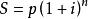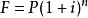# 2020年度银行校招常见经济金融知识——复利2年年末的终值=1*(1+10%)=(1+10%)

2年年末存入一元

3年年末的终值=1*(1+10%)^2+1*(1+10%)=(1+10%)^2+(1+10%)

3年年末存入一元

4年年末的终值=1*(1+10%)^3+1*(1+10%)^2+1*(1+10%)=(1+10%)^3+(1+10%)^2+(1+10%)

4年年末存入一元

5年年末的终值=1*(1+10%)^4+1*(1+10%)^3+1*(1+10%)^2+1*(1+10%)=(1+10%)^4+(1+10%)^3+(1+10%)^2+(1+10%)

5年年末存入一元　年金终值F=(1+10%)^4+(1+10%)^3+(1+10%)^2+(1+10%)+1

F=A+A×(1+i)^1+…+A×(1+i)^(n-1)，

F=A[1-(1+i)^n]/[1-(1+i)]

F=A[1-(1+i)^n]/[1-1-i]

F=A[(1+i)^n-1]/i　式中[(1+i)^n-1]/i的为普通年金终值系数、或后付年金终值系数，利率为i，经过n期的年金终值记作(F/A，i,n),可查普通年金终值系数表。